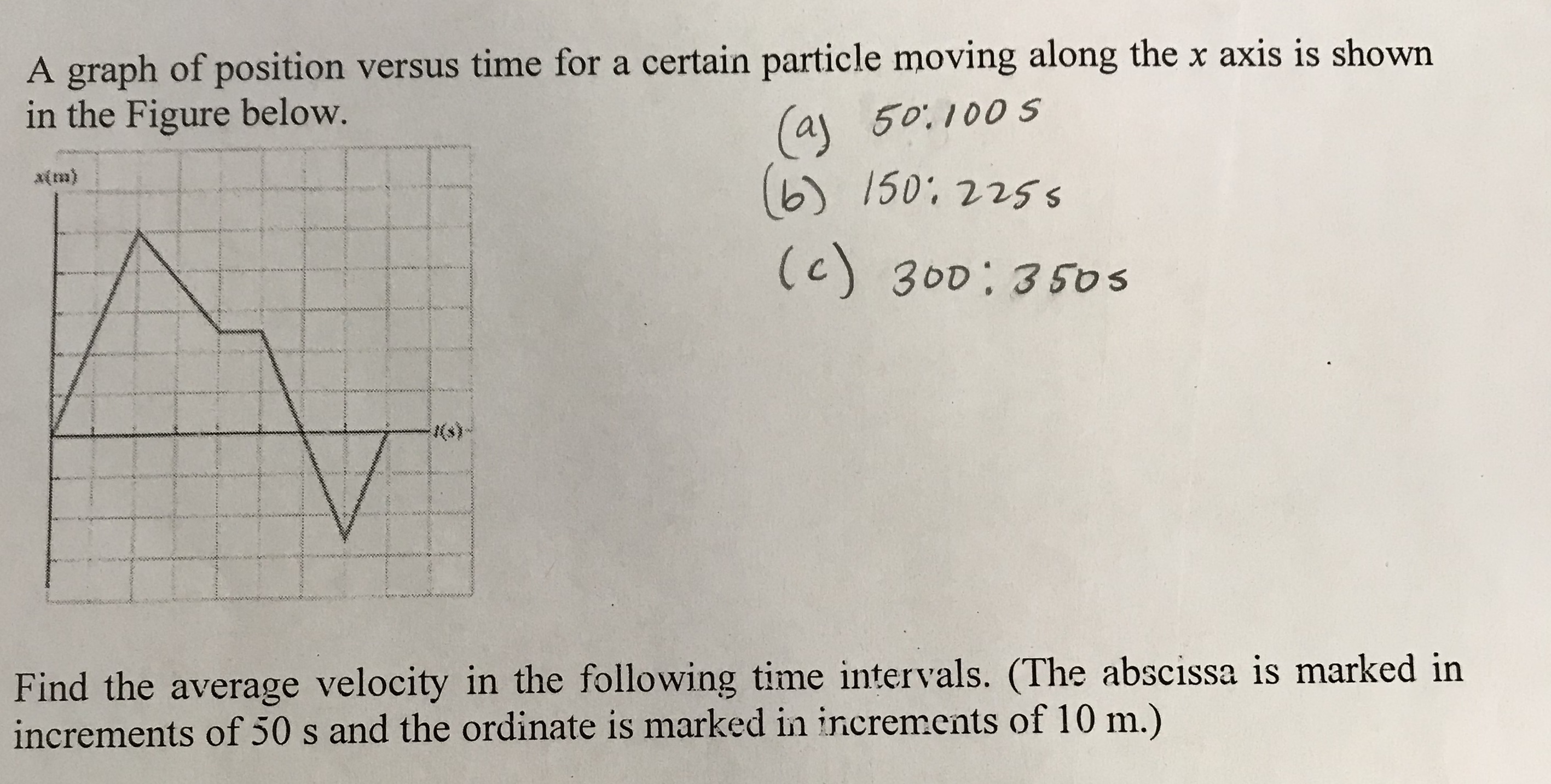# A graph of position versus time for a certain particle moving along the x axis is shownin the Figure belowa 50. 100 sb 150: 225 sFind the average velocity in the following time intervals. (The abscissa is marked inincrements of 50 s and the ordinate is marked in increments of 10 m.)

Question
4 views

I am confused on the formula for Average velocity I believe I got it right I’m just trying to be accurate. Here is the problem. Part a, b and c are written in pencil that need to be solved. Thank you.help_outlineImage TranscriptioncloseA graph of position versus time for a certain particle moving along the x axis is shown in the Figure below a 50. 100 s b 150: 225 s Find the average velocity in the following time intervals. (The abscissa is marked in increments of 50 s and the ordinate is marked in increments of 10 m.) fullscreen
check_circle

Step 1

The equation for the average velocity is given by,

Step 2

The same equation can be used to determine the aver...

### Want to see the full answer?

See Solution

#### Want to see this answer and more?

Solutions are written by subject experts who are available 24/7. Questions are typically answered within 1 hour.*

See Solution
*Response times may vary by subject and question.
Tagged in

### Kinematics